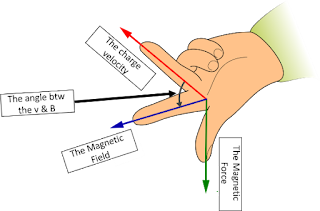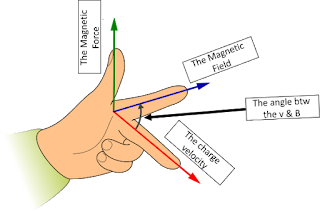# The Magnetic Fields

A negative charge generates an electric field.
A moving charge
generates a magnetic field.

### What do we mean by permanent magnet and how it is generated?

The magnet is a magnetic field that is caused by the individual electrons in the last orbit of some atoms.

#### The Electron orbits

2. About itself (called a spinning motion). This rotation generates a very small magnetic field.

#### Atoms with even number of electrons in their last orbit do not generate a magnetic field because:

(Electrons in each state rotate opposite to each other, so they cancel each other out)

### Magnet types

1. Permanent magnet is Fe3O4.
2. Temporary magnets are Materials that are magnetized under the influence of another magnetic field and for a specified period of time (magnetization goes away with the removal of the affecting field).

3. A magnet generated by an electrical current.

### Angle " θ " Cases:

 The Case What does it mean? if θ = 0 àsin 0 = 0 àFB = 0 The charge is moving parallel or opposite to the direction of "B" if θ = 90 à sin 90 = 1 à FB = qvB FB is perpendicular to the plane containing the magnetic field "B" and charge velocity "v" θ May be any value other than 0 or 90

### FB direction varies according to charge type: (when θ = 90 )

 If the charge is negative (electron) à the FB is negative and its direction is downward If the charge is positive (proton) à the FB is positive and its direction is upward Upside-Down Left Hand Rule Left Hand Rule### The magnetic field "B":

We can infer the law of the magnetic field "B" from the law of magnetic force "FB"